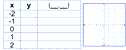# 5th Grade Math Worksheets: Geometry

These geometry worksheets review 2-D geometry including classifying angles, triangles and quadrilaterals, calculating perimeters and areas and working with circles. 3D geometry is introduced with rectangular prisms.  Reading and plotting points on a coordinate grid is also covered.

Classify angles
Acute, obtuse, right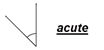Measure and classify angles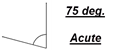Estimating angles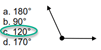Classify triangles
Equilateral, isosceles, scalene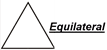Square / rectangle/ rhombus / parallelogram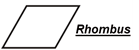## Area & perimeter

Area / perimeter problems for rectangles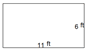Area / perimeter of irregular rectangular shapes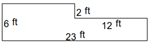Area of right triangles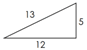Area of triangles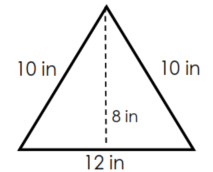Area of triangles, parallelograms and trapezoids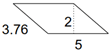## Circles

Circumference of a circle

Area of a circle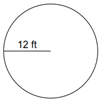## 3D: Volume & Surface Area

Rectangular prisms & cubes - missing sides

Volume & surface area of rectangular prisms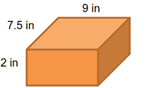## Nets & models

Nets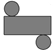3D Models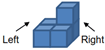## Coordinate Grids

Plot points on a coordinate grid

Read the coordinates of points on a grid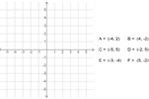Translations on a coordinate plane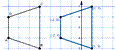Plot functions on the coordinate plane